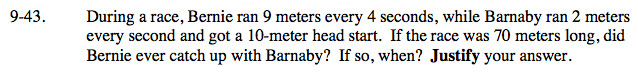### Home > AC > Chapter 9 > Lesson 9.2.2 > Problem9-43

9-43.Define variables and write an equation for Bernie and an equation for Wendel.
x = time in seconds
y = distance in meters

$\text{Bernies's rate = \frac{9\ meters}{4\ seconds}}$

$y=\frac{9}{4}x$

$\text{Barnaby's rate = \frac{2\ meters}{1\ second}\ \ \ \ \ 10 meter headstart = starting point}$

y = 2x + 10

To catch up, their equations would need to be equal.

Solve for x.

$\frac{9}{4}x=2x+10$

x = 40 seconds

Substitute x into one of the original equations and find y.
If the distance (y) is less than 70, Bernie caught up with Barnaby.

y = 90 meters
Bernie can't catch up with Barnaby in 70 meters.

Use the eTool below to graph the equations for Bernie and Barnaby.
Look at your graph to determine if Bernie ever caught up with Barnaby and if so, when.
Click on the link at right for the full eTool version: AC 9-43 HW eTool